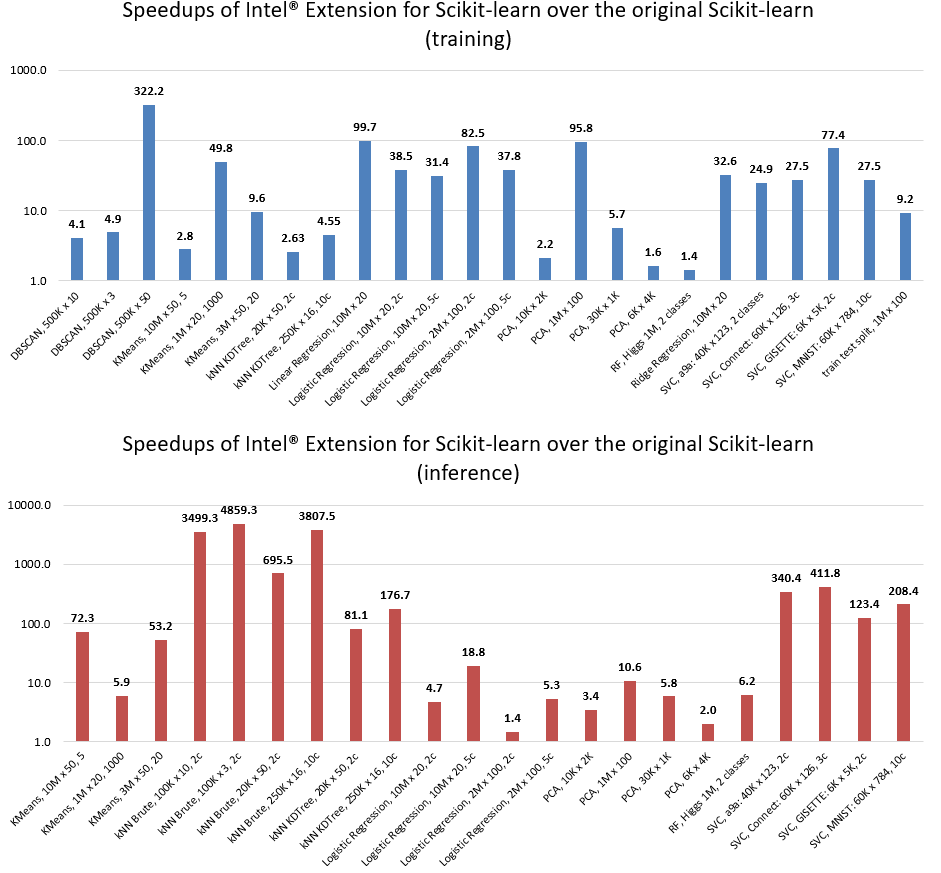「scikit-learn」作为经典的机器学习框架，其运算速度一直广受用户的诟病。今天分享一个新包 「sklearnex」，可以在不改变原有代码的基础上，获得数十倍甚至上千倍的效率提升。

## 安装sklearnex#

pip3 install notebook scikit-learn-intelex


## 快速上手#

### scikit-learn原代码#

import numpy as np
from sklearn.cluster import KMeans

X = np.array([[1,  2], [1,  4], [1,  0],
[10, 2], [10, 4], [10, 0]])

kmeans = KMeans(n_clusters=2, random_state=0).fit(X)
print(f"kmeans.labels_ = {kmeans.labels_}")


Run

kmeans.labels_ = [1 1 1 0 0 0]


### 加速代码#

import numpy as np
from sklearnex import patch_sklearn
patch_sklearn() #启动加速补丁

# 加速补丁放置于sklearn之前
from sklearn.cluster import KMeans

X = np.array([[1,  2], [1,  4], [1,  0],
[10, 2], [10, 4], [10, 0]])
kmeans = KMeans(n_clusters=2, random_state=0).fit(X)
print(f"kmeans.labels_ = {kmeans.labels_}")


Run

kmeans.labels_ = [1 1 1 0 0 0]


### 去掉加速#

from sklearnex import unpatch_sklearn

unpatch_sklearn()

# Re-import scikit-learn algorithms after the unpatch:
from sklearn.cluster import KMeans


## 效率对比#